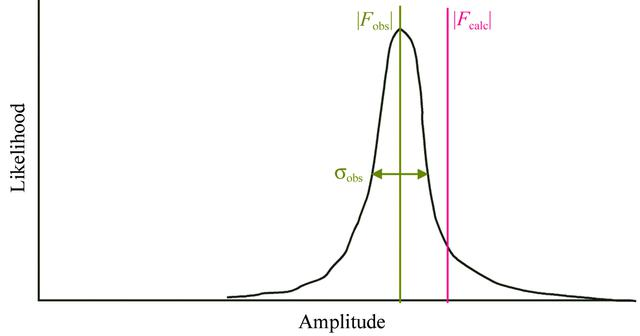disable zoom     view article Figure 3 Probability distribution for one reflection in the least-squares worldview. In least-squares analysis it is assumed that the observed and calculated structure factors have exactly the same phase, so the only error to consider is in the magnitude of the observation. The true value of |Fobs| is assumed to be represented by a one-dimensional Gaussian centered at its measured value and with a spread related to its estimated standard uncertainty, σobs. The calculated amplitude is assumed to have no spread at all. In this example, the parameters of the model should be modified to cause |Fcalc| to decrease.BIOLOGICALCRYSTALLOGRAPHY
ISSN: 1399-0047
Volume 60| Part 12| November 2004| Pages 2156-2168